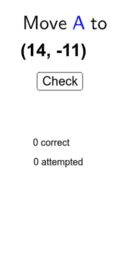Plotting Points Practice

A set of similar applets suitable for independent practice plotting randomly generated points on a coordinate plane/grid with feedback. The number of attempts and number correct, as well as percent correct out of the number attempted, are shown. Includes a reset button to start over.
•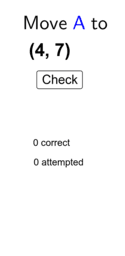Plotting Points Practice: quadrant I, whole numbers 0 to 12

•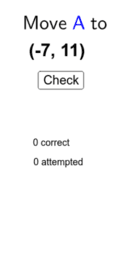Plotting Points Practice: quadrants I&II, integers -12 to 12

•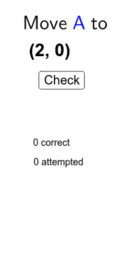Plotting Points Practice: 4 quadrants, integers -4 to 4

•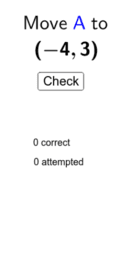Plotting Points Practice: 4 quadrants,integers -4 to 4 by .5

•